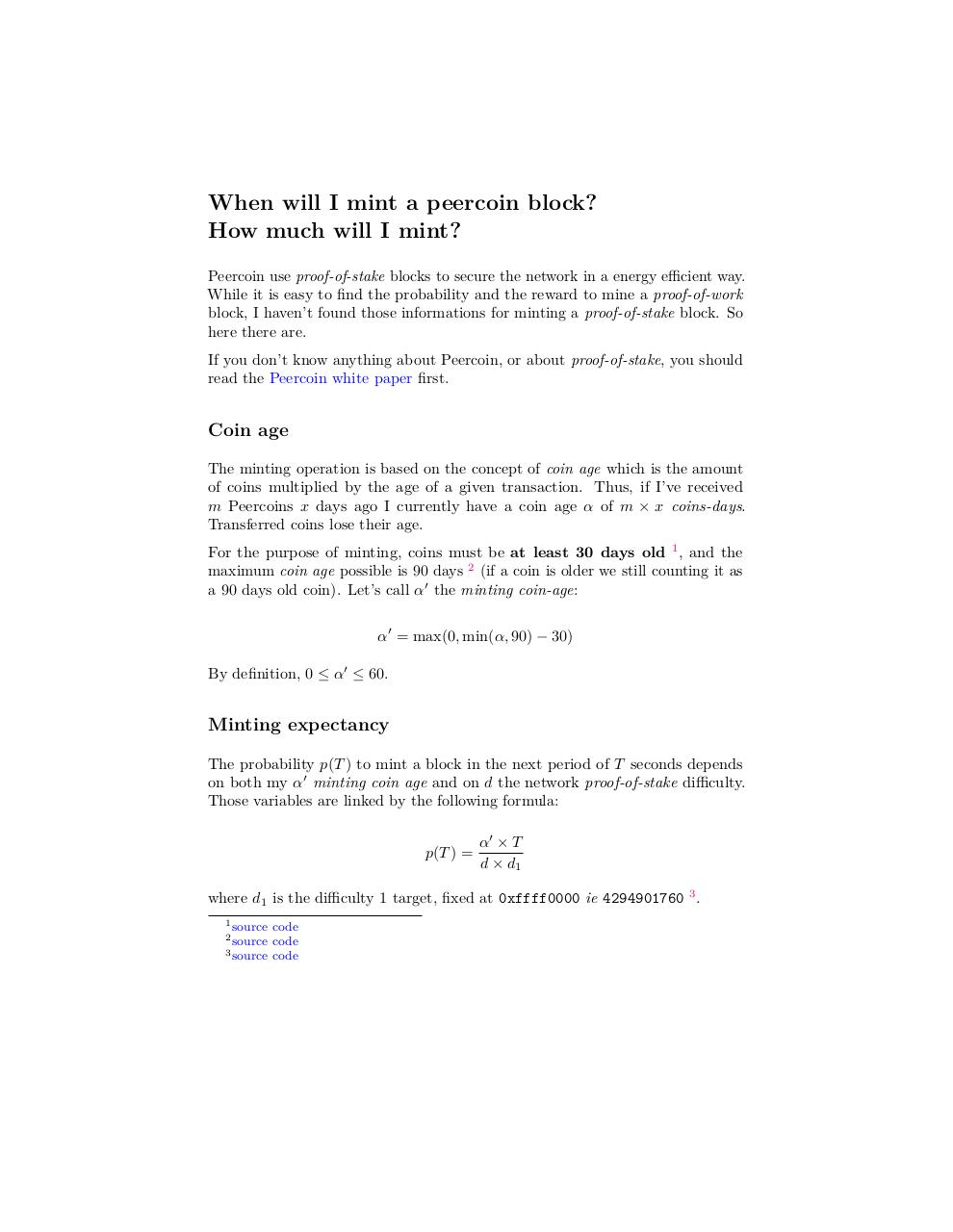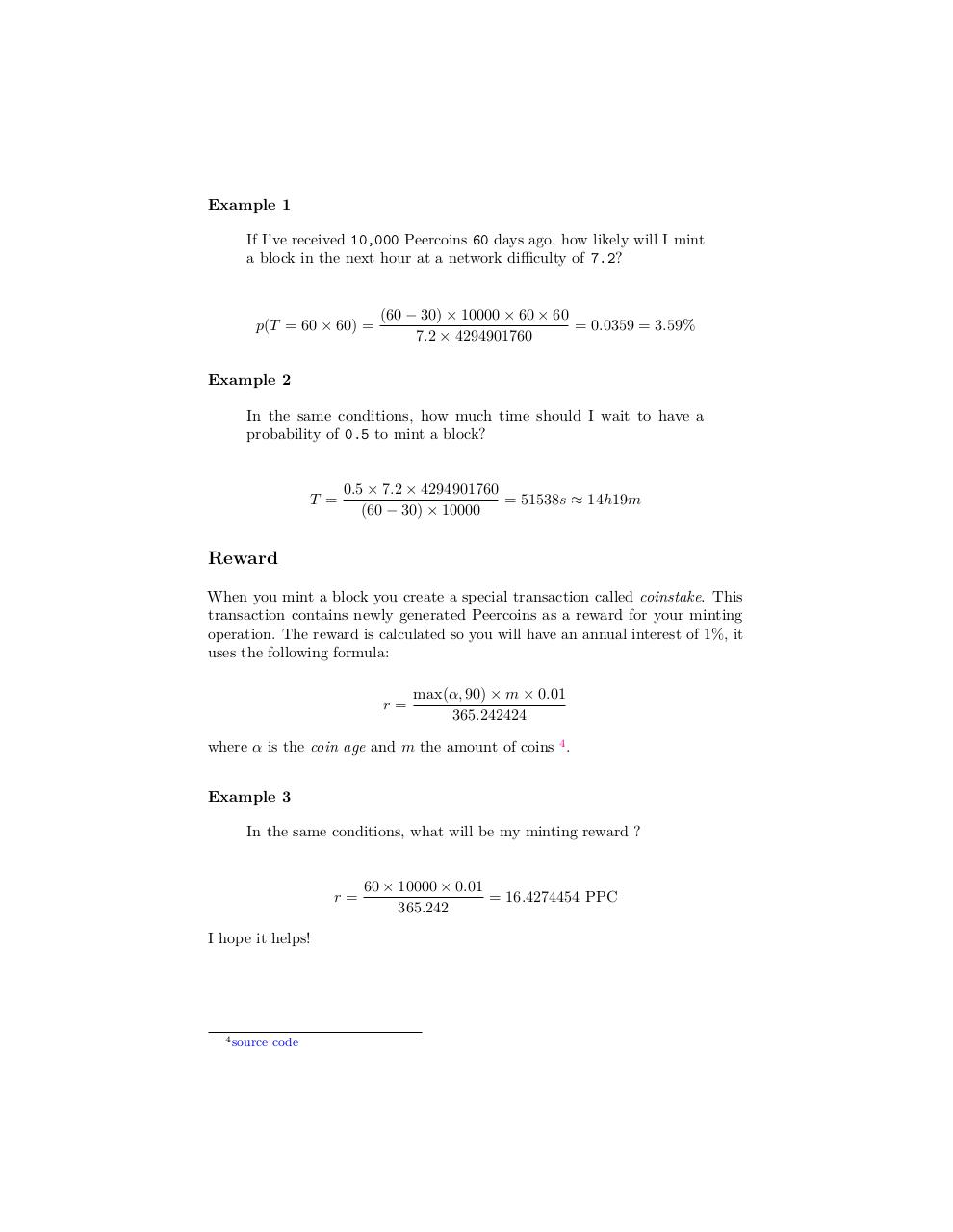# ppcmint .pdf

### File information

Original filename: ppcmint.pdf

This PDF 1.5 document has been generated by LaTeX with hyperref package / pdfTeX-1.40.14, and has been sent on pdf-archive.com on 13/02/2014 at 20:27, from IP address 82.240.x.x. The current document download page has been viewed 851 times.
File size: 187 KB (2 pages).
Privacy: public file

ppcmint.pdf (PDF, 187 KB)

### Document preview

When will I mint a peercoin block?
How much will I mint?
Peercoin use proof-of-stake blocks to secure the network in a energy efficient way.
While it is easy to find the probability and the reward to mine a proof-of-work
block, I haven’t found those informations for minting a proof-of-stake block. So
here there are.
If you don’t know anything about Peercoin, or about proof-of-stake, you should
read the Peercoin white paper first.

Coin age
The minting operation is based on the concept of coin age which is the amount
of coins multiplied by the age of a given transaction. Thus, if I’ve received
m Peercoins x days ago I currently have a coin age α of m × x coins-days.
Transferred coins lose their age.
For the purpose of minting, coins must be at least 30 days old 1 , and the
maximum coin age possible is 90 days 2 (if a coin is older we still counting it as
a 90 days old coin). Let’s call α0 the minting coin-age:
α0 = max(0, min(α, 90) − 30)
By definition, 0 ≤ α0 ≤ 60.

Minting expectancy
The probability p(T ) to mint a block in the next period of T seconds depends
on both my α0 minting coin age and on d the network proof-of-stake difficulty.
Those variables are linked by the following formula:
p(T ) =

α0 × T
d × d1

where d1 is the difficulty 1 target, fixed at 0xffff0000 ie 4294901760 3 .
1 source

code
code
3 source code
2 source

Example 1
If I’ve received 10,000 Peercoins 60 days ago, how likely will I mint
a block in the next hour at a network difficulty of 7.2?

p(T = 60 × 60) =

(60 − 30) × 10000 × 60 × 60
= 0.0359 = 3.59%
7.2 × 4294901760

Example 2
In the same conditions, how much time should I wait to have a
probability of 0.5 to mint a block?

T =

0.5 × 7.2 × 4294901760
= 51538s ≈ 14h19m
(60 − 30) × 10000

Reward
When you mint a block you create a special transaction called coinstake. This
transaction contains newly generated Peercoins as a reward for your minting
operation. The reward is calculated so you will have an annual interest of 1%, it
uses the following formula:
r=

max(α, 90) × m × 0.01
365.242424

where α is the coin age and m the amount of coins 4 .
Example 3
In the same conditions, what will be my minting reward ?

r=
I hope it helps!

4 source

code

60 × 10000 × 0.01
= 16.4274454 PPC
365.242#### HTML Code

Copy the following HTML code to share your document on a Website or Blog

#### QR Code### Related keywords